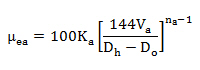# Effective Viscosity Calculation

The viscosity of drilling mud will change with a change in the shear rate because in some degree drilling fluids are shear-thinning. Because of its nature of drilling mud, people create the new term of viscosity called “Effective Viscosity” to compensate the change in shear rate of viscosity. By definition, the effective viscosity means the viscosity of Newtonian fluid that gives the same shear stress at the same shear rate.

The following equation is the effective viscosity equation.Where

µea = effective viscosity in the annulus, centi-poise

Ka = consistency factor in the annulus, poise

Dh = hole diameter, inch

Do = Outside diameter of pipe, inch

na = power law constant of drilling mud in the annulus

Va = annular velocity in the annulus, ft/sec

Please see the following example for more understanding.

Ka = 6.63 poise

Dh = 8.5 inch

Do = 5 inch

na = 0.514

Va = 7 ft/secµea = 42.53 centi-poise

Reference:Drilling Hydraulic Books

Share the joyWorking in the oil field and loving to share knowledge.

### 2 Responses to Effective Viscosity Calculation

1.kresimer zubac says:

what is consistency factor in the annulus ? how to calculate it?
thanks

•DrillingFormulas.Com says:

You should get from the mud report.

This site uses Akismet to reduce spam. Learn how your comment data is processed.